Next: Optimization Examples Up: Optimization - General Bound Previous: Placing and Tightening the

# General Bound Maximization and Properties

General bound maximization refers to the use of a bound along with some important manipulation techniques to reduce intractable optimization problems into more reasonable forms. We define a set of manipulations to facilitate bounding for a wide variety of functions f which may contain all sorts of arithmetic operations, power terms, and elementary functions. These manipulations can be applied iteratively and recursively to ultimately break down a complicated function into several simple bounds. These bounds can then be reassembled and used to perform a simple maximization as in the previous example, moving the current operating point x1 to a better (higher) locus on the function, x2 and iterating until convergence.

• Property 1 - Addition and Subtraction

If a function involves a summation, the individual components of the function can be bounded separately and then the bounds can be summed to form a bound on the overall function (Equation 6.11).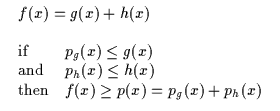(6.11)

For subtraction, we can just define h(x) as -h(x) and bound that quantity with ph(x).

• Property 2 - Multiplication and Division

If a function involves a multiplication, the logarithm of the multiplied terms can be bounded and then the resulting bounds can again be summed to form a bound on the overall function (Equation 6.12). Since logarithms are being used, assume that f,g,h are positive functions. Here we specifically want to rearrange the bounding to be a sum of bounds for simplicity. Otherwise, it could be possible to just multiply two bounds and still have an overall bounding.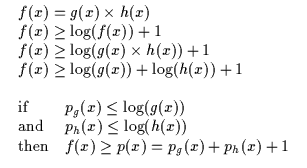(6.12)

For division, we can just define log(h(x)) as -log(h(x)) and bound that quantity with ph(x).

• Property 3 - Composition

If an expression involves the composition of two functions, the expression as a whole can be bounded by the composing a bound on one function with the other sub-function. (Equation 6.13).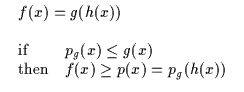(6.13)

For example, consider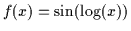where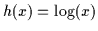and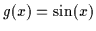. Assume that we can bound g with a parabola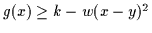. Then function f(x) can be bounded by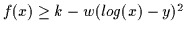. It is far simpler to take derivatives of the bounding function and set these to zero to iteratively optimize fthan it is to directly solve for the maximum via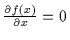.

• Property 4 - Partial Linearity

If a function involves a linear operation then its bounds can also be modified linearly as long as the scale factors are non-negative (Equation 6.14).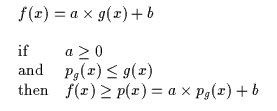(6.14)

In addition, a scaling operation on the domain can also be applied to the bound as well (Equation 6.15).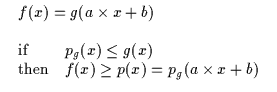(6.15)

• Property 5 - Jensen's Inequality

A well known tool for bounding techniques is Jensen's inequality which is repeated below for convenience (Equation 6.16).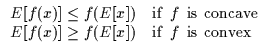(6.16)

• Property 6 - Function Bounding

Often, it is useful to replace a function in an expression with a lower bounding function which makes contact with it at the current operating point. Figure 6.5 depicts how the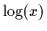function can be replaced by upper bounds. In this figure, the current operating point is x*=1. The convex functions depicted are p1(x)=x-1,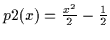, and p3(x)=e(x-1)-1. This property will be very important later as we apply these techniques to conditional density estimation.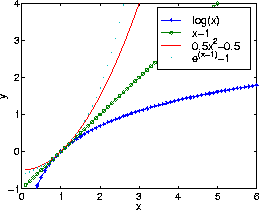• Property 7 - Monotonic Transformations

Of course, there are many possible bounds to select for any optimization and it is difficult to find a single form for the bound which will always work. This is true even if we have a rather generic form such as a parabola. For instance, it is impossible to lower bound f(x) = -x4 with a quadratic since the function will always be decreasing faster than any parabola. However, for many functions, it is possible to get around this by instead maximizing another function, say h(x) = g(f(x)) where g is a monotonically increasing function such as g(x) = ex which, when composed with f will have the same maxima and minima as f but will behave in a more controllable way. For this example, h(x) = ef(x) = e-x4 results and this can be bounded with a parabola. Selecting the monotonic function to use in these situations is often intuitively obvious.Next: Optimization Examples Up: Optimization - General Bound Previous: Placing and Tightening the
Tony Jebara
1999-09-15